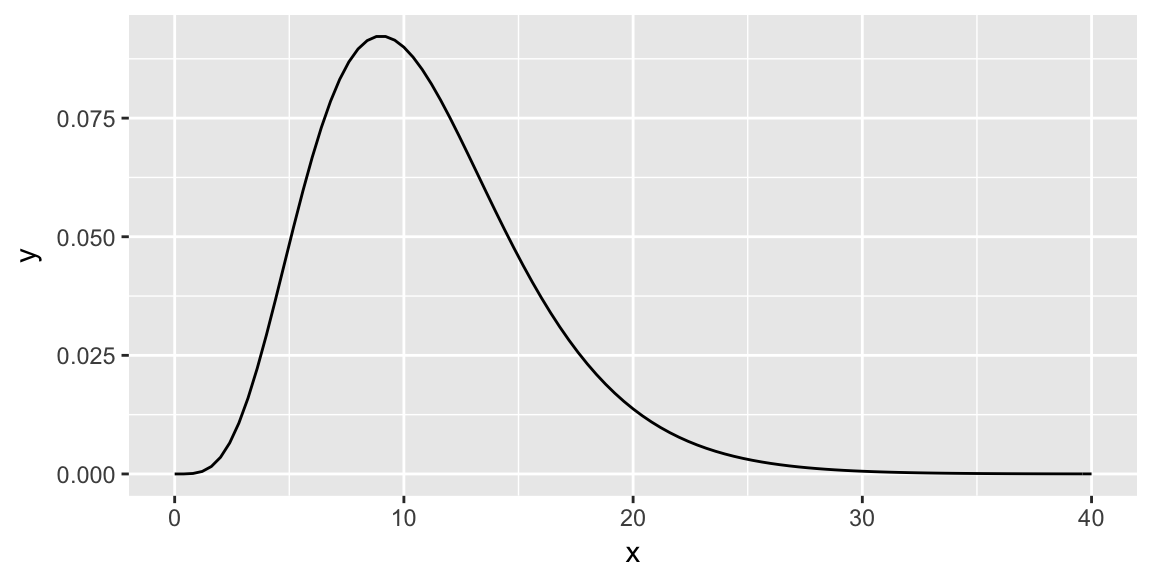# So far …

## Tests of center

Test/Procedure Parameter Setting
Z-test Population mean
t-test Population mean
Binomial exact test Population proportion (mean)
Binomial z-test Population proportion (mean)
Sign test Population median
Signed Rank test Population mean/median

## Two tests of scale

1. Chi-square test of variance

2. t-test of variance

# Chi-square test of variance

## Chi-square test of variance

Population: $$Y \sim$$ some population distribution

Sample: n i.i.d from population, $$Y_1, \ldots, Y_n$$

Parameter: Population variance $$\sigma^2 = Var(Y)$$

## Sample variance

The sample variance, $s^2 = \frac{1}{n-1}\sum_{i= 1}^n \left(Y_i - \overline{Y} \right)^2$

is an unbiased and consistent estimate of $$\sigma^2$$.

Furthermore, if $$Y \sim N(\mu, \sigma)$$, it can be shown the sampling distribution of $$s^2$$ is a scaled Chi-square distribution: $(n-1)\frac{s^2}{\sigma^2} \sim \chi^2_{(n-1)}$

## Using the sampling distribution to formulate a test

Assume the population distribution is $$N(\mu, \sigma^2)$$.

Consider the null hypothesis $$H_0: \sigma^2 = \sigma^2_0$$.

Let the test statistic be: $X(\sigma_0^2) = (n-1)\frac{s^2}{\sigma_0^2}$ What’s the distribution of the test statistic if the null hypothesis is true?

## Rejection regions

For a test at level $$\alpha$$:

• $$H_A: \sigma^2 > \sigma_0^2$$: Reject $$H_0$$ if $$X(\sigma_0^2) >$$
• $$H_A: \sigma^2 < \sigma_0^2$$: Reject $$H_0$$ if $$X(\sigma_0^2) <$$
• $$H_A: \sigma^2 \ne \sigma_0^2$$: Reject $$H_0$$ if $$X(\sigma_0^2) >$$
or $$X(\sigma_0^2) <$$

Shade the area for the p-value when $$X(\sigma_0) = 20$$, with $$H_A: \sigma^2 > \sigma_0^2$$Shade the area for the p-value when $$X(\sigma_0) = 20$$, with $$H_A: \sigma^2 < \sigma_0^2$$Shade the area for the p-value when $$X(\sigma_0) = 20$$, with $$H_A: \sigma^2 \ne \sigma_0^2$$## p-values: In general

p-value is:

• $$H_A: \sigma^2 > \sigma_0^2$$:
• $$H_A: \sigma^2 < \sigma_0^2$$:
• $$H_A: \sigma^2 \ne \sigma_0^2$$:

where $$X \sim \chi^2_{(n-1)}$$

In R: $$P(X \le x)$$ = pchisq(x, df = n - 1)

## Confidence interval

From inverting test statistic.

$$(1 - \alpha)100$$% confidence interval

$\left( \frac{s^2(n - 1)}{\chi^2_{(n-1)}(1 - \alpha/2)} , \frac{s^2(n - 1)}{\chi^2_{(n-1)}(\alpha/2)}\right)$

## What if the population isn’t Normal

(from Sarah Emerson’s slides F2016)# t-test of variance

## t-test of variance

An alternative to the Chi-square test of variance, based on considering a transformed response:

$Z_i = \left( Y_i - \overline{Y}\right)^2 \quad i = 1, \ldots, n$

What is $$E(Z)$$, are the $$Z_i$$ independent?

## A CLT for the sample variance

The $$Z_i$$ aren’t independent but are weakly dependent, turns out there is a CLT for this case, as long as $$Z$$ has finite fourth moment:

$\frac{\overline{Z} - E(Z)}{\sqrt{Var(Z)/n}} \rightarrow_d N(0, 1)$

Substitute in for $$E(Z)$$ $\frac{\overline{Z} - \frac{n - 1}{n}\sigma^2}{\sqrt{Var(Z)/n}} \rightarrow_d N(0, 1)$

We don’t know the population variance of the $$Z$$ (transformed $$Y$$), so substitute sample estimate for it.

Under null hypothesis $$H_0: \sigma^2 = \sigma_0^2$$

$t(\sigma_0^2) = \frac{\overline{Z} - \frac{n-1}{n} \sigma_0^2 }{\sqrt{s_{Z}^2/n}} \, \dot \sim \, t_{(n-1)}$

So to test the null $$H_0: \sigma^2 = \sigma_0^2$$, do a t-test on $$Z_i = \left(Y_i - \overline{Y}\right)^2$$ with the null hypothesis $$H_0: \mu_Z = \frac{n-1}{n}\sigma_0^2$$.

## Performance of t-test of variance

Charlotte’s simulations, rejection rate of $$H_0$$ for $$\alpha = 0.05$$.

50 500 5000
Chi-square(10) 0.12 0.069 0.055
Exp(1) 0.177 0.084 0.072
t(5) 0.148 0.076 0.068
Uniform(0, 1) 0.057 0.057 0.042